# Automatic Generation Control of Multi-area Interconnected Power Systems Using ANN Controller

Automatic Generation Control of Multi-area Interconnected Power Systems Using ANN Controller

University of Quebec (ETS), 1100 Rue Notre-Dame Quest, Montreal Québec H3C 1K3, Canada

Universiti Tenaga Nasional, 43000 Kajang, Selangor, Malaysia

Concordia University, 1250 Guy St, Montreal, Quebec H3H 2L3, Canada

Ericsson, GAIA Department, 8275 Trans Canada Route, saint-Laurent, Quebec H4S 0B6, Canada

Corresponding Author Email:
Khaled.alzaareer.1@ens.etsmtl.ca
Page:
1-10
|
DOI:
https://doi.org/10.18280/ria.340101
1 October 2019
|
Accepted:
20 December 2019
|
Published:
29 February 2020
| Citation

OPEN ACCESS

Abstract:

Load as well as power flow in tie-line are continuously varying in interconnection power systems. This paper presents an efficient method based on artificial intelligence control for automatic generation control (AGC) of a three-area power network. The control method implements Artificial Neural Network (ANN) to damp the frequency deviation and the fluctuation in the tie line power caused by load disturbances. The performance of the proposed controller is compared with classical control methods (PI and PID). The results showed that ANN-based control method is more efficient than others approaches. In this paper, MATLAB/SIMULINK package is used to investigate the results.

Keywords:

automatic generation control, PI controller, PID controller, artificial neural network controller (ANN), tie line, Area Control Error (ACE), MATLAB/SIMULINK

1. Introduction

With the development of interconnected power system, the necessity for automatic generation control has been raised in power system design and operation . In real power systems, the load demands are continuously and randomly varying. When such a disturbance is occurred on power system, tie-line power interchange and area frequency change too.

The ability to meet load changes (i.e. disturbances) by action of generators is weak. This is due to the physical consideration which can cause unbalance between the actual generation power and the scheduled one .

Since the interconnected power systems require operating at constant frequency with adequate and consistent electric power, an automatic generation controller design is needed to eliminate the variations in system frequency and sustain the power transfer between areas .

Automatic Generation Control (AGC) can be defined as a scheduling process for the power outputs by system generators within a particular area as a reaction for any change in network frequency, tie line transfer power in order to keep them within predetermined limits [4, 5].

Several control strategies have been developed in order to keep the network frequency and tie-line transfer power within acceptable limits. The most common controller employed for AGC is based on classical control theory. These conventional controllers can be found in the researches [6-9]. These studies observed that an integral controller is very simple to implement and can provide zero steady state deviation. However, this kind of controller gives poor dynamic response.

However, power systems operate at different operating conditions. The current operating condition continuously varies according to demand amounts of consumers while the gain values of the conventional controllers are constants. This makes the conventional controllers are not valid for different operating conditions. Moreover, the goal of Automatic Generation Control is to keep the steady state errors at zero and to keep the transient behavior of the network stable. Therefore, Artificial Intelligent (AI) controllers are more suitable for that improvement. Recently, Different artificial intelligence-based methods have been proposed in the literature. Fuzzy control methods [10-12], bacterial foraging-based optimization technique , and neural networks [3, 14, 15] are applied to AGC problem.

In this work, a comparison between the Artificial Neural Network based control and the classical control for automatic generation control is investigated.

2. Frequency Response Modeling

To study the dynamic behavior of power system, the mathematical modeling of each of the components of power system is needed. One of the most common methods used in the modeling is the transfer function method.

2.1 Generator model

The model of any generator dynamic can be done by using swing equation given in Eq. (1).

$\frac{2 H}{\omega_{S}} \frac{d^{2} \Delta \delta}{d t^{2}}=\Delta P_{m}-\Delta P_{e}$   (1)

With speed expressed in per unit and by taking Laplace transform of Eq. (1), we obtain:

$\Delta \omega(s)=\frac{1}{2 H S}\left[\Delta P_{m}(s)-\Delta P_{e}(s)\right]$   (2)

where, Pm represents the mechanical powers, Pe is the electrical powers, ω represents the angular velocity, and H represents the inertia constant of the generators. Eq. (2) can be represented by a block diagram illustrated in Figure 1.

## 1.jpg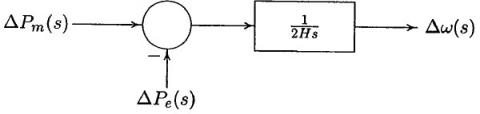Figure 1. Transfer function diagram of generator

The relation between the speed and load can be expressed by Eq. (3).

$\Delta \mathrm{P}_{\mathrm{e}}=\Delta \mathrm{P}_{\mathrm{L}}+D \Delta \omega$  (3)

where, ΔPL represents the non-frequency sensitive load change and DΔω represents the frequency sensitive load change. D represents the percentage change in load divided by the percentage change in frequency. Eq. (3) can be represented by the block diagram illustrated in Figure 2.

## 2.jpg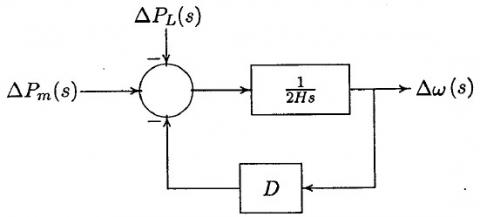Figure 2. Transfer function diagram of generator and load

Thus, the combined function for the models of both generator and load can be seen in Figure 3.

## 3.jpgFigure 3. Combined transfer function diagram of generator and load

2.3 Prime mover model

Turbines models relate the changes in mechanical power outputs ΔPm, to the changes in steam valve positions ΔPv as shown in Eq. (4). Modeling the prime mover of the nonreheat steam turbines can be represented with a single time constant TT, as shown in the block diagram shown in Figure 4.

$G_{T}(s)=\frac{\Delta P_{m}(s)}{\Delta P_{v}(s)}=\frac{1}{1+\tau_{T} s}$   (4)

## 4.jpg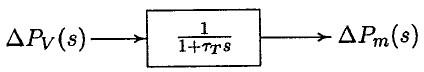Figure 4. Transfer function diagram of nonreheat steam turbine

2.4 Governor model

The governor can be considered as a comparator with an output ΔPg which represents the difference between the reference set point ΔPref and the power $\frac{\Delta \omega}{\mathrm{R}}$ as shown in Eq. (5). The output ΔPg can be transferred by an amplifier to the steam valve position command ΔPv.

If we assume that the relation is linear with a simple time constant Tg, Eq. (6) is obtained. The model of the speed governor of steam turbine cab be seen in Figure 5.

$\Delta P_{g}(s)=\Delta P_{r e f}(s)-\frac{1}{R} \Delta \omega(S)$  (5)

$\Delta \mathrm{P}_{\mathrm{v}}(\mathrm{s})=\frac{1}{\mathrm{R}+\tau_{g}} \Delta \mathrm{P}_{\mathrm{g}}(\mathrm{s})$  (6)

## 5.jpg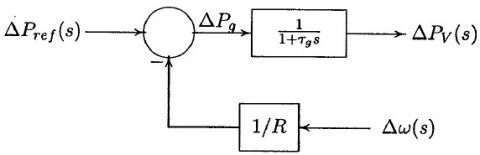Figure 5. Transfer function diagram of governor system steam turbine

By combining all the block diagrams of all components, we can obtain the overall block diagram of load-frequency control of a power network as presented in Figure 6.

## 6.jpgFigure 6. Load frequency control diagram of an isolated power system

When system load changed, the turbine speed is also changed. At this moment the governor can change the input of the steam to a new value to keep a constant system speed. However, the constant speed will not be the set point, and there will be an offset. So, an integral controller is needed to restore the speed or the frequency to the normal value by adjusting the generation automatically as shown in Figure 7. This process is called Automatic Generation Control.

## 7.jpgFigure 7. AGC for an isolated power system

3. Automatic Generation Control of an Interconnected Power System

Any interconnected power network consists of multi control areas. It is assumed that all generators in a power system can act as a coherent group . This means that each area can be replaced by an equivalent turbine, generator and governor system. Three areas interconnected in ring topology have been considered in this paper as shown in Figure 8.

## 8.jpg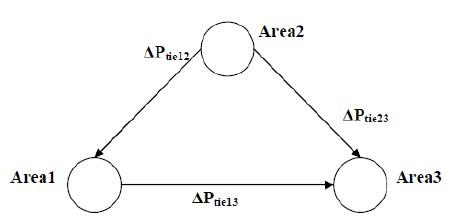Figure 8. Ring topology connected three area model

During normal operation, the real power transferred over tie-line is represented by Eq. (7):

$P_{12}=\frac{V_{1} V_{2}}{X_{12}} \sin \left(\delta_{1}-\delta_{2}\right)$  (7)

where, X12 represents the tie-line reactance, δ1 and δ2 represent the power angles of equivalent machines. V1 and V2 represent the voltages at equivalent machines of the terminals of area 1 and area 2, respectively. By linearizing Eq. (9) for a small deviation in tie line flow ΔP12 about an equilibrium point δ10 δ20, we obtain the tie power deviation represented in Eq. (8):

$\Delta P_{12}=P s_{12}\left(\Delta \delta_{1}-\Delta \delta_{2}\right)$  (8)

where, Ps is called synchronizing power coefficient and can be specify by Eq. (9).

$P s=\left.\frac{d P_{12}}{d \delta_{12}}\right|_{\delta_{12} \circ}=\frac{\left|E_{1}\right|\left|E_{2}\right|}{X_{12}} \cos \left(\delta_{1}^{\circ}-\delta_{2}^{\circ}\right)$  (9)

Considering the relation between area power angle δ and speed ω, Eq. (8) can be written as Eq. (10).

$\Delta P_{12}=P_{S_{12}}\left(\int \Delta \omega_{1}-\int \Delta \omega_{2}\right)$  (10)

where, Δω1 and Δω2 are speed deviations in area 1 and area 2, respectively. By taking the Laplace transform of (10), we obtain Eq. (11). Thus, the block diagram of a tie- line is represented in Figure 9.

$\Delta P_{12}(s)=\frac{P s_{12}}{S}\left(\int \Delta \omega_{1}(s)-\int \Delta \omega_{2}(s)\right)$  (11)

## 9.jpg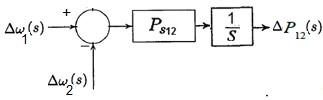Figure 9. Block diagram representation of a tie-lie

Similarly, the change in tie line power between area 1 and area 3 is illustrated in Eq. (12).

$\Delta P_{13}(s)=\frac{P s_{13}}{S}\left(\int \Delta \omega_{1}(s)-\int \Delta \omega_{3}(s)\right)$  (12)

Considering (11) and (12), the total variation in tie line transfer power between area 1 and the other two areas can be obtained as Eq. (13).

$\Delta P_{1}=\Delta P_{12}+\Delta P_{13}$$=\frac{1}{S}\left[\sum_{j=2,3} P s_{1 j} \Delta \omega_{1}-\sum_{j=2,3} P s_{1 j} \Delta \omega_{j}\right] (13) For N control areas, the power change can be calculated as in (14). \Delta P_{i}=\sum_{j=1 \atop j \neq i}^{N} \Delta P_{i j}$$=\frac{1}{S}\left[\sum_{j=1 \atop j \neq i}^{N} P s_{i j} \Delta \omega_{i}-\sum_{j=1 \atop j \neq i}^{N} P s_{i j} \Delta \omega_{j}\right]$  (14)

The change in the tie line transfer power for a particular area is caused as a result for load variation of that area. Thus, the tie-line power deviation ΔPi can be added to the mechanical power change (ΔPm,i ) and area load disturbance (ΔPL) using an appropriate sign.

In a multi-area power system, the system should maintain frequency or speed at its normal value, and maintains the tie line transfer power within acceptable limits. In addition, each area should absorb its own load schedule . This is generally achieved by adding a tie line power deviation to the deviation of the system frequency in the supplementary feedback loop. This process is called the tie-line bias control. A combination between frequency and tie line power changes for area 1 is called as the area control error (ACE). ACE for any area i can be represented by Eq. (15).

$A C E_{i}=\sum_{i=1}^{n} \Delta P_{i j}+K_{i} \Delta \omega$  (15)

where, Ki is called area bias which determines the amount of interaction during a disturbance in other areas. A steady state performance is achieved when the value of the gain Ki is chosen such that it equals to the frequency bias factor of that area Bi which identify by Eq. (16).

$B_{i}=\frac{1}{R_{i}}+D_{i}$  (16)

This control can adjust the reference power set point (ΔPref) automatically, and when steady state is reached, this control drives ACEs to zero. Thus, the system frequency and the tie-lie power are restored to the normal values. Furthermore, since all areas are in energy balance, any load changes must have been absorbed within each area. Figure 10 shows the AGC for three area power systems.

## 10.jpg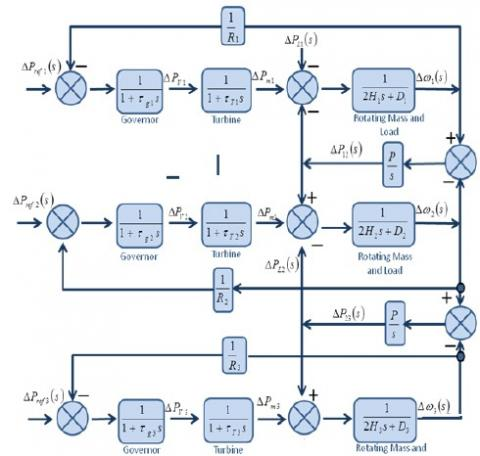Figure 10. AGC for three area power system

4. ANN Controller

Artificial neural networks are an information processing system. ANN has elements called as neurons which process the data and information. In this method, the signals are transferred by using links. These links are also assigned with weights. The weights are multiplied with the input signals for a reprehensive neural net. The final output signals are resulted by performing activations to the net input .

The ANN control presented in this work is the NARMA L2 controller. The calculation needed for this type of control is very small. The NARMA L2 controllers are normally designed to eliminate the nonlinearities and the dynamic behaviors of a non linear systems. It has the ability to change the nonlinear dynamic systems into implicit algebraic models. This means that this controller able to control the trajectory of the any system. The Structure of NARMA-L2 Neural controller is presented in Figure 11.

## 11.jpg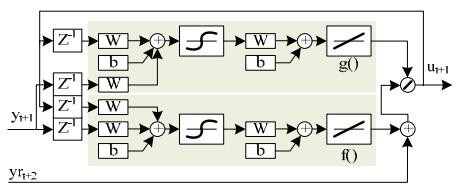Figure 11. The structure of NARMA L2 controller

The NARMA L2 Neural controller normally has two sub-networks, f and g. To build up the neural controller, one needs to choose the number of delayed inputs and outputs according to the structural models. There is also a need to choose the size of the hidden layers. This size is selected such that we ensure that the system can find an approximation for the nonlinearity of the system, in accurate way. NARMA L2 controller can simply rearrange the model of the neural network plants which can be trained offline in batch form using set of input output data pairs to find an approximation for the nonlinear functions f and g .

Once the nonlinear function of f and g are modeled, the controller then rearrange of the two sub-networks of the plant model in an easy way to obtain the control. The closed loop system of this method is presented in Figure 12 .

In the work, the system frequency deviation, tie line transfer power deviations and load disturbance are selected as inputs for ANN control.

## 12.jpgFigure 12. Closed Loop system of NARMA-L2 Controller

5. Simulation Results

In this study, the AGC of an interconnection three area power network is used. Three types of controls have been presented for AGC control. These controls are based on PI, PID and ANN controllers. A comparison between the performances of these controller is done.  MATLAB/ SIMULINK software is used to investigate the results. For each scenario, the responses of system frequency, mechanical power, and tie line transfer power deviations are achieved.

5.1 Uncontrolled system

The Simulink model of uncontrolled AGC is shown in Figure 13. For 1% step load disturbance, the frequency, mechanical power, and tie-line power deviation are shown in Figure 14. It can be observed from the results that power system is highly oscillated with the latest settling time. It also can be shown that steady state error does not equal to zero.

## 13.jpg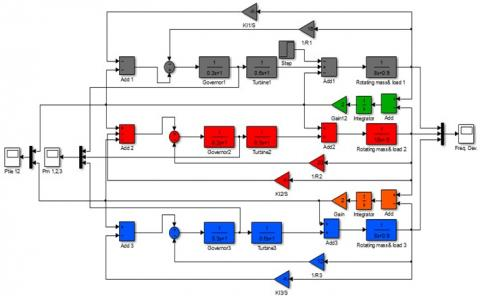Figure 13. Simulink model of uncontrolled AGC of three area power system

## 14a.jpg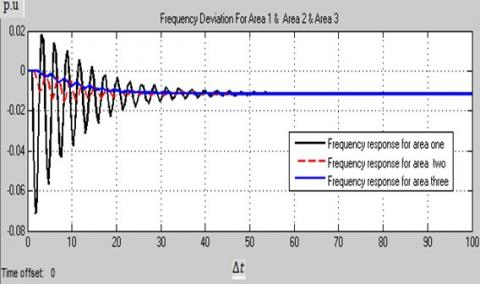(a)

## 14b.png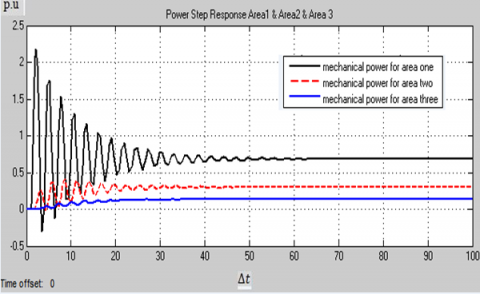(b)

## 14c.jpg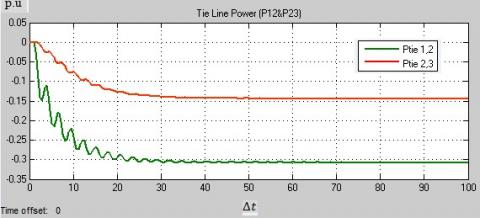(c)

Figure 14. (a) Frequency deviation (b) mechanical power deviation (c) tie-line power deviation step response of uncontrolled AGC of three area power system

5.2 PI controller

The Simulink model of AGC with PI controller can be seen in Figure 15. For 1% step load disturbance, the integrator gain is adjusted for a satisfactory response. As we can see from Figure 16, the deviation in the system frequency goes to zero with a settling time less than at uncontrolled case. Also, the tie-line transfer power deviation is reduced to zero. It is also clear that the increase in the load of area1 has been met by an increasing in generation of area 1.

## 15.jpg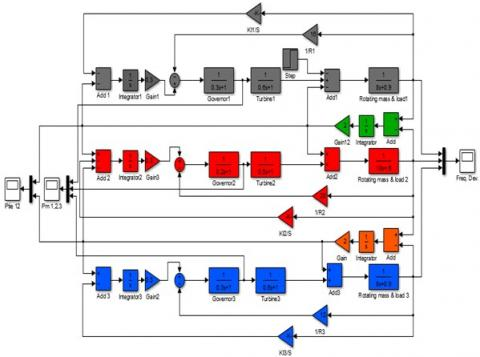Figure 15. Simulink model of AGC with PI controller of three area power system

## 16a.jpg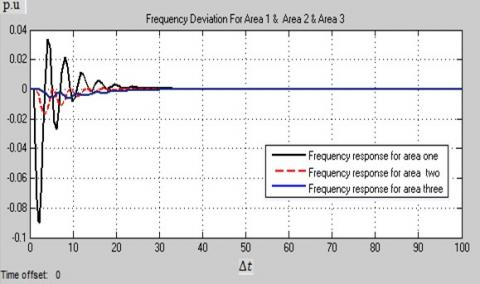(a)

## 16b.jpg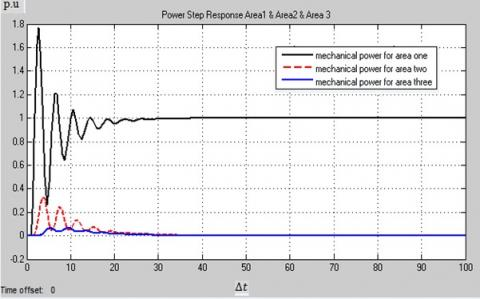(b)

## 16c.jpg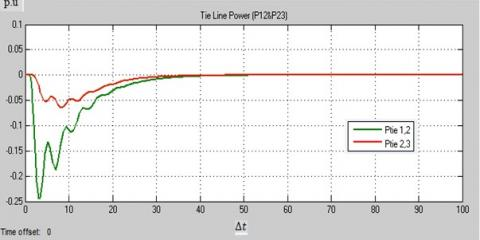(c)

Figure 16. (a) Frequency deviation (b) mechanical power deviation (c) tie-line power deviation step response of AGC with PI controller of three area power system

5.3 PID controller

The Simulink model of AGC with PID controller can be seen in Figure 17. For 1% step load disturbance, the frequency, mechanical power, and tie line transfer power deviation are illustrated in Figure 18. The PID gains are adjusted for a satisfactory response. It can be observed that the deviation in the system frequency goes to zero with a lower settling time. Also, we can see that the deviation in the tie-line transfer power reduces to zero. It is also clear that the increase in the load of area1 has been met by an increasing in generation of area 1. It is worth mentioning that a PID tuner developed in MATLAB is used for PID parameters tuning in this paper. It can compute a linearized plant model seen by the controller. It automatically identifies the plant input and output, and uses the current operating point for the linearization.

## 17.jpg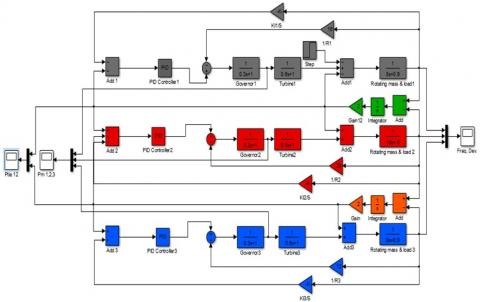Figure 17. Simulink model of AGC with PID controller of three area power system

## 18a.jpg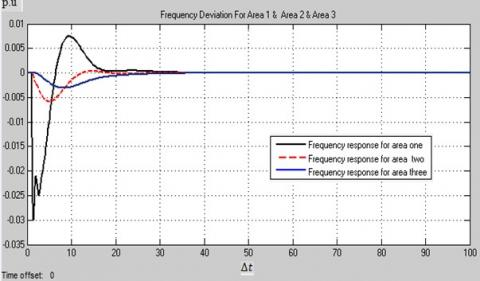(a)

## 18b.jpg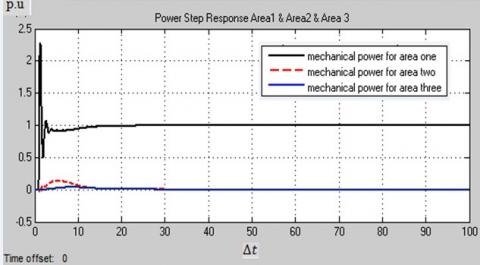(b)

## 18c.jpg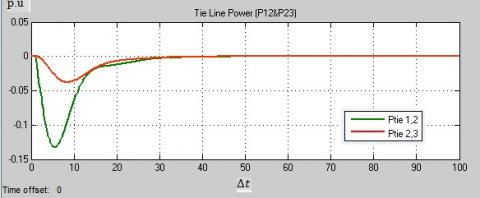(c)

Figure 18. (a) Frequency deviation (b) mechanical power deviation (c) tie-line power deviation step response of AGC with PID controller of three area power system

## 19.jpg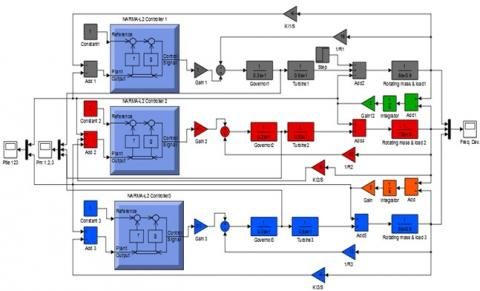Figure 19. Simulink model of AGC with ANN controller of three area power system

5.4 ANN controller

The Simulink model of AGC with NARMA-L12 controller is shown in Figure 19. The neural networks are trained in batch form using Levenberg-Marquardt algorithm.  For 1% step load disturbance, the frequency, mechanical power, and tie line transfer power deviation are shown in Figure 20.

It can be observed that the deviation in the system frequency goes to zero with an earlier settling time. Moreover, we can see that the deviation in the tie line power change reduces to zero. Moreover, the increase in the load of area1 has been met by an increasing in generation area 1.

## 20a.jpg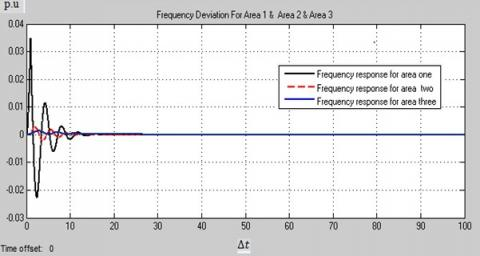(a)

## 20b.jpg(b)

## 20c.jpg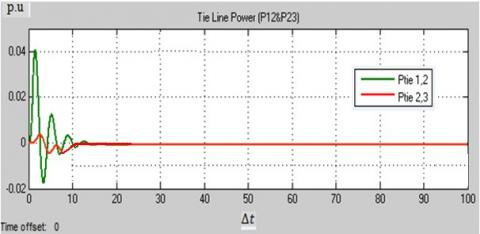(c)

Figure 20. (a) Frequency deviation (b) mechanical power deviation (c) tie-line power deviation step response of AGC with ANN controller of three area power system

## 21.jpg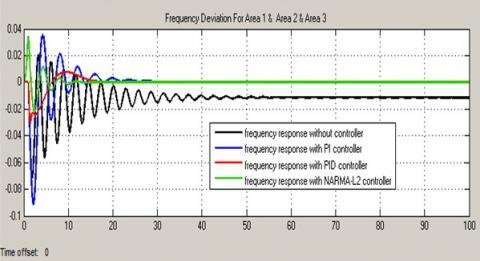Figure 21. Frequency deviation in area 1 by comparison of PI, PID, and ANN controllers

5.5 Comparison of PI, PID, and ANN controllers

The changes in frequency in area 1 in response to step load disturbance in a three area power system with conventional PI controller, PID controller, ANN controller are shown in Figure 21. It shows that ANN controller gives better dynamic responses.

6. Conclusions

In this work, an efficient method based on ANN method is presented for AGC problem of a three-area power network.  A comparison with other classical controllers (PI and PID) is done to illustrate the dynamic performances of the controllers. Transient behavior of the frequency, mechanical output power, and tie-line power flows are investigated in accordance with 1% load disturbance using MATLAB/Simulink package. As illustrated in the simulation and analysis, results conduct the following points.

The results showed that the response of the PI-controlled power network is highly oscillated with latest settling time compared to other controlled systems. Performance comparison shows that the response of the PID-controlled power system is the lowest oscillated response with shorter settling time compared to PI controller and a longer settling time as compared to ANN controller. The results also show that the response of the ANN controlled power system is lower oscillated response with the earliest settling time. The responses with the ANN based control show that the performance of ANN controller is better than the classical controllers. It can be observed that ANN controllers can effectively improve the damping of system oscillations. Tables 1 & 2 shows the comparative study of settling time and peak overshot, respectively. The results of these tables show that ANN controller has a smaller settling time and peak overshoot than the other controller.

As a result, ANN controllers are advance adaptive control configurations. It can provide faster controls than other methods.

Our future work is to develop a technique to optimize the hyperparameter in ANN. Moreover, other approaches from deep neural networks research, such as ReLU activate function will be used to test ANN controller.

Table 1. Comparative study of settling time

 Controller Δf1  (sec) Δf2 (sec) Δf3 (sec) ΔP12 (sec) ΔP23 (sec) PI 33 21 30 40 50 PID 28 20 27 35 35 ANN 14 12 12 17 37

Table 2. Comparative study of peak overshoot
 Controller Δf1  (p.u) Δf2 (p.u) Δf3 (p.u) ΔP12 (p.u) ΔP23 (p.u) PI 0.09 0.017 0.005 0.24 0.07 PID 0.03 ,0.006 0.003 0.135 0.035 ANN 0.03 0.002 0.001 0.04 0.007
Appendix

The typical values of system parameters for the nominal operating condition are as follows:

 Parameter Names Area 1 Area 2 Area 3 Governor Speed Regulation R1= 16 R2= 20 R3=12 Frequency Sensitive Load Coefficient D1= 0.9 D2=0.6 D3=0.9 Inertia Constant H1=8 H2=10 H3=8 Governor Time Constant τg1= 0.3s τg2=0.2s τg3=0.3 s Turbine Time Constant τT1= 0.6s τT2=0.5s τT3=0.6s Synchronizing Power Coefficient P12 = 2 s P23=2s P31=2s
References

 Kundur, P. (1994). Power System Stability and Control. McGraw-Hill Education, New York.

 Prakash, S., Sinha, S.K., Pandey, A.S., Singh, B. (2009). Impact of slider gain on load frequency control using fuzzy logic controller. ARPN Journal of Engineering and Applied Sciences, 4(7).

 Patel, N., Jain, B.B. (2014). Automatic Generation control of four area power systems using Ann controllers. International Journal of Electrical, Electronics and Computer Engineering, 3(1): 62-68.

 Saadat, H. (2002). Power System Analysis. 6th edition, Tata McGraw-Hilledition.

 IEEE Committee Report. (1970). IEEE standard definitions of terms for automatic generation control of electric power system. IEEE Transactions on Power Apparatus and Systems, PAS-89, pp. 1358-1364.

 Prakash, S., Sinha, S.K. (2010). Artificial intelligent & PI in load frequency control of interconnected power system. International Journal of Computer Science & Emerging Technologies, 1(4): 377-384.

 Khan, C.M., Konar, S.C., Chanda, C.K. (2014). Study of thermal generating units of multi-area power system connected to grid and its stability. International Journal of Recent Development in Engineering and Technology, 2(2): 55-65.

 Nagendra, M., Krishnarayalu, M.S. (2012). PID controller tuning Simulink for multi area power systems. International Journal of Engineering Research & Technology, 1(7).

 Khodabakhshian, A., Hooshmand, R. (2010). A new PID controller design for automatic generation control of hydro power systems. Electrical Power and Energy Systems, 32(5): 375-382. https://doi.org/10.1016/j.ijepes.2009.11.006

 Zenk, H., Akpınar, A.S. (2012). Multi zone power systems load-frequency stability using fuzzy logic controllers. Journal of Electrical and Control Engineering, 2: 49-54.

 Nanda, J., Mangla, A. (2004). Automatic generation control of an interconnected hydro-thermal system using conventional integral & fuzzy logic controller. IEEE International Conference on Electric Utility Deregulation Reconstructing & Power Technology, Hong Kong, China, 1: 372-377. https://doi.org/10.1109/DRPT.2004.1338524

 Talaq, J., Al-Basri, F. (1999). Adaptive fuzzy gain scheduling for load frequency control. IEEE Transaction on Power System, 14(1): 145-150. https://doi.org/10.1109/59.744505

 Nanda, J., Mishra, S., Saikia, L.C. (2009). Maiden application of bacterial foraging-based optimization technique in multiarea automatic generation control. IEEE Transactions on Power Systems, 24(2): 602-609. https://doi.org/10.1109/TPWRS.2009.2016588

 Demiroren, A., Zeynelgil, H.L., Sengor, N.S. (2001). The application of ANN technique to load-frequency control for four-area power system. IEEE Porto Power Tech Conference, PPT001, Porto, Portugal.

 Demiroren, A., Zeynelgil, H.L., Sengor, N.S. (2001). The application of ANN technique to load-frequency control for three-area power system. IEEE Porto Power Tech Conference, PPT001, Porto, Portugal. https://doi.org/10.1109/PTC.2001.964793

 Ngia, L.S.H., Sjöberg, J. (2000). Efficient training of neural nets for nonlinear adaptive filtering using a recursive Levenberg-Marquardt algorithm. IEEE Transactions on Signal Processing, 48(7): 1915-1927. https://doi.org/10.1109/78.847778

 Pukrittayakame, A., De Jesus, O., Hagan, M.T. (2002). Smoothing the control action for NARMA-L2 controllers. The 2002 45th Midwest Symposium on Circuits and Systems, 2002. MWSCAS-2002., Tulsa, OK, USA, pp. III-III. https://doi.org/10.1109/MWSCAS.2002.1186964